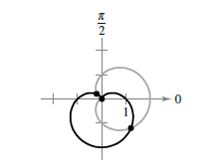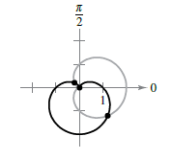Chapter 10.5, Problem 27E

Chapter
Section
Textbook Problem

# Finding Points of Intersection In Exercises 27-34, find the points of intersection of the graphs of the equations. r = 1 + cos θ r = 1 − sin θTo determine

To Calculate: The intersection points of the graph of polar curves r=1+cosθ,r=1sinθ.Explanation

Given:

Formula Used:

r=1+cosθ,r=1sinθ

Calculation:

The Polar equations are: r=1+cosθ,r=1sinθ

Solve simultaneously and get,

1+cosθ=1sinθ

cosθ=sinθ

tanθ=1

θ=3π4,7π4

Replace r by r and

### Still sussing out bartleby?

Check out a sample textbook solution.

See a sample solution

#### The Solution to Your Study Problems

Bartleby provides explanations to thousands of textbook problems written by our experts, many with advanced degrees!

Get Started

#### Find more solutions based on key concepts